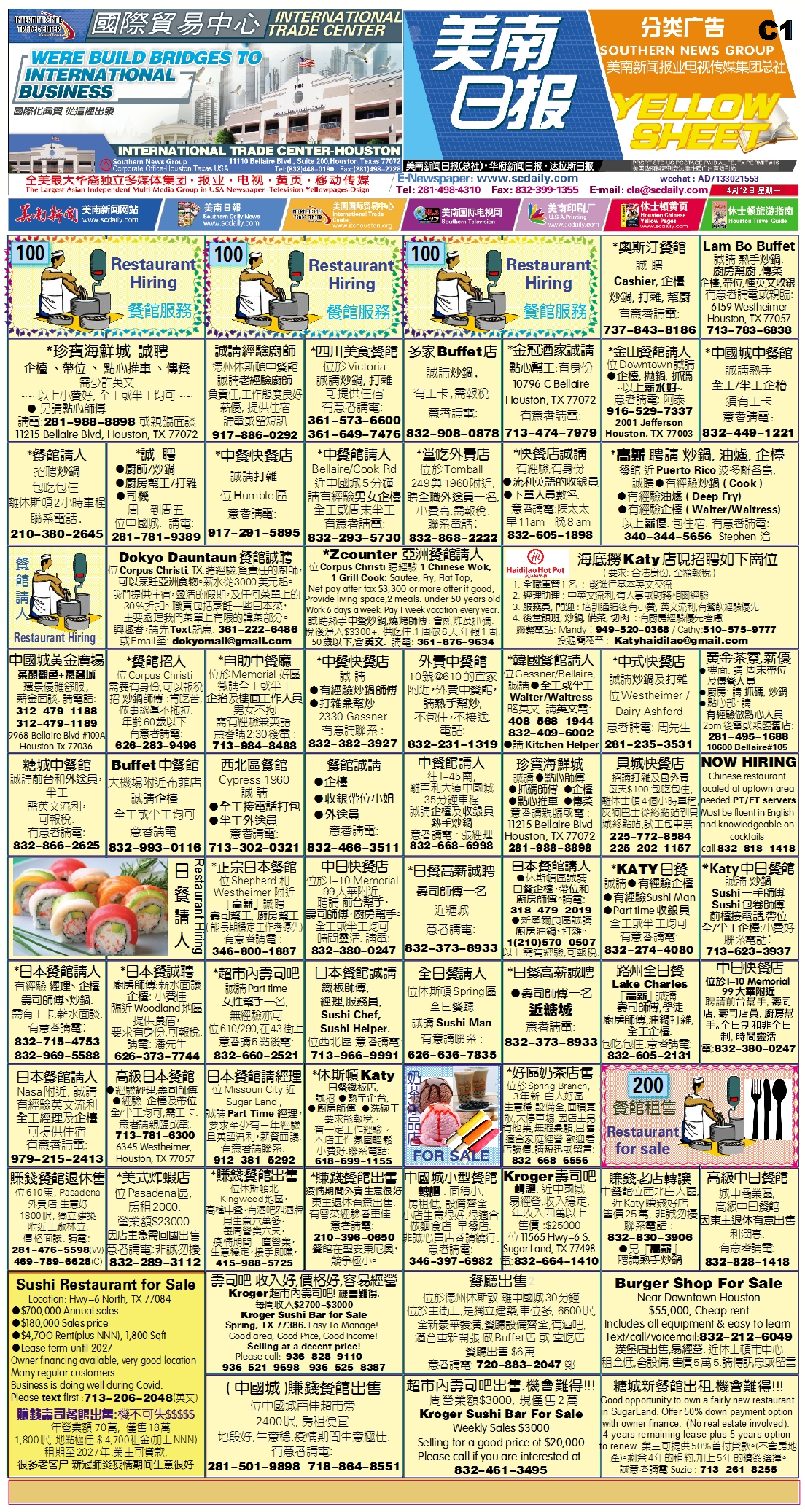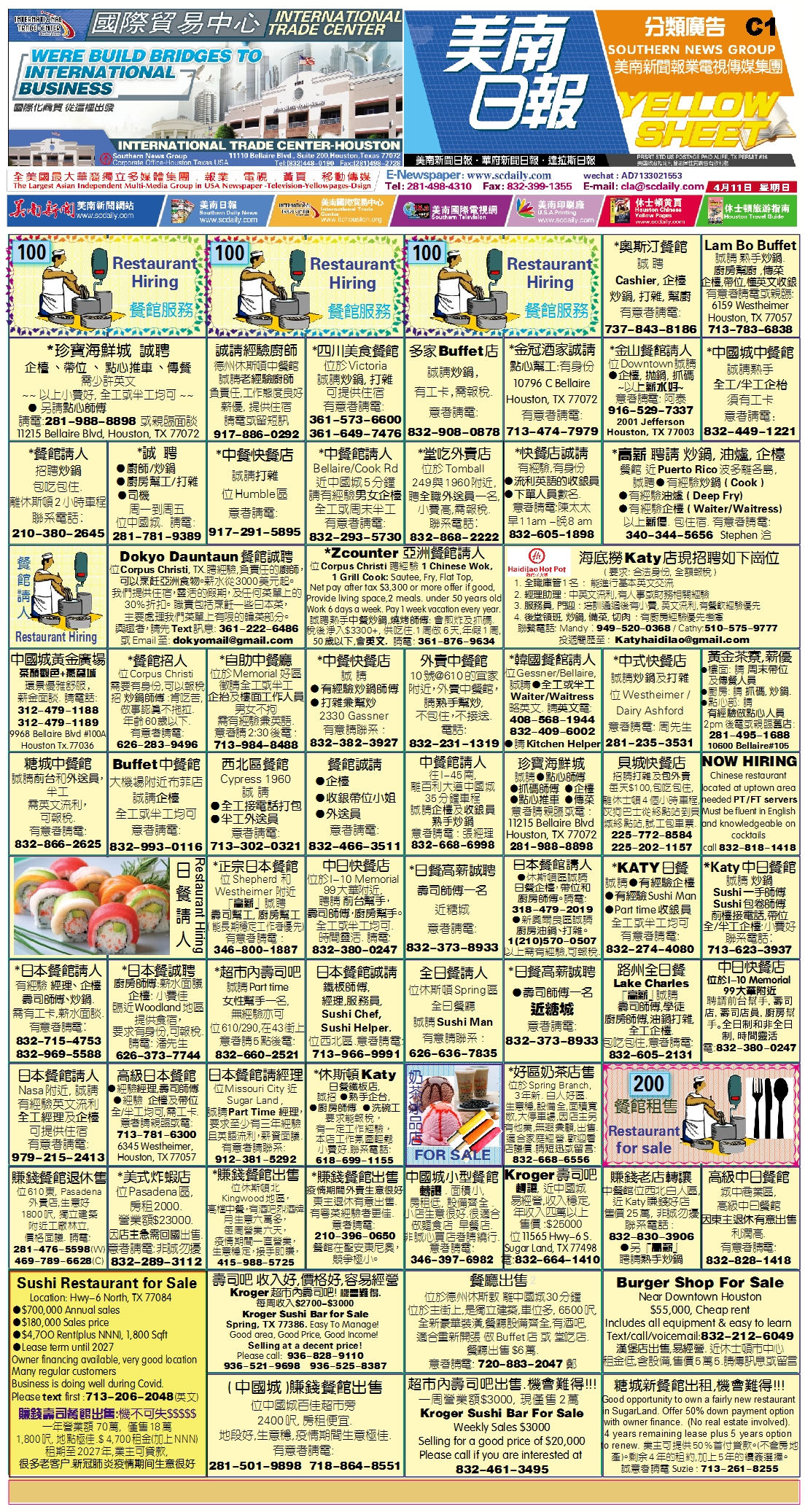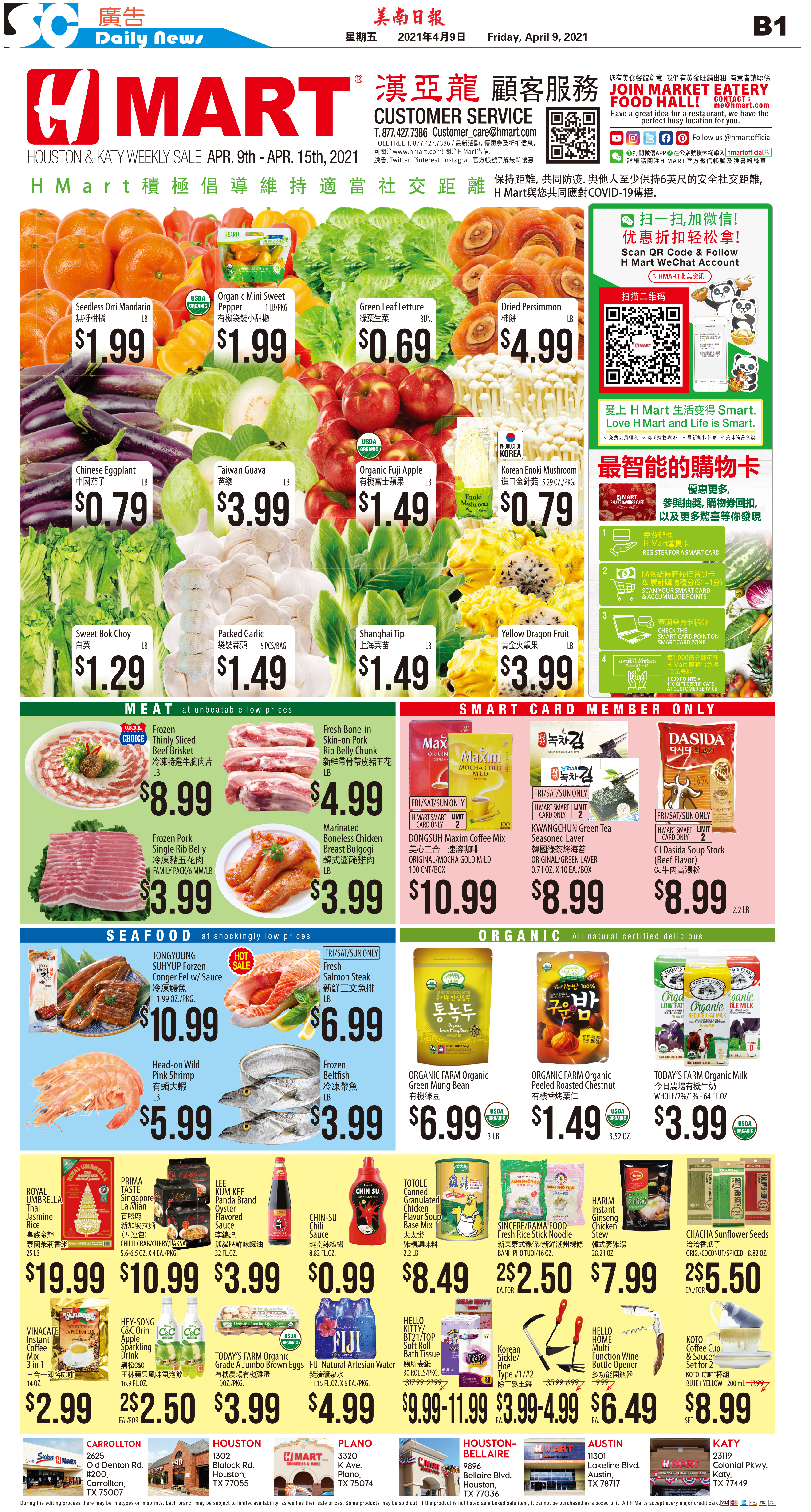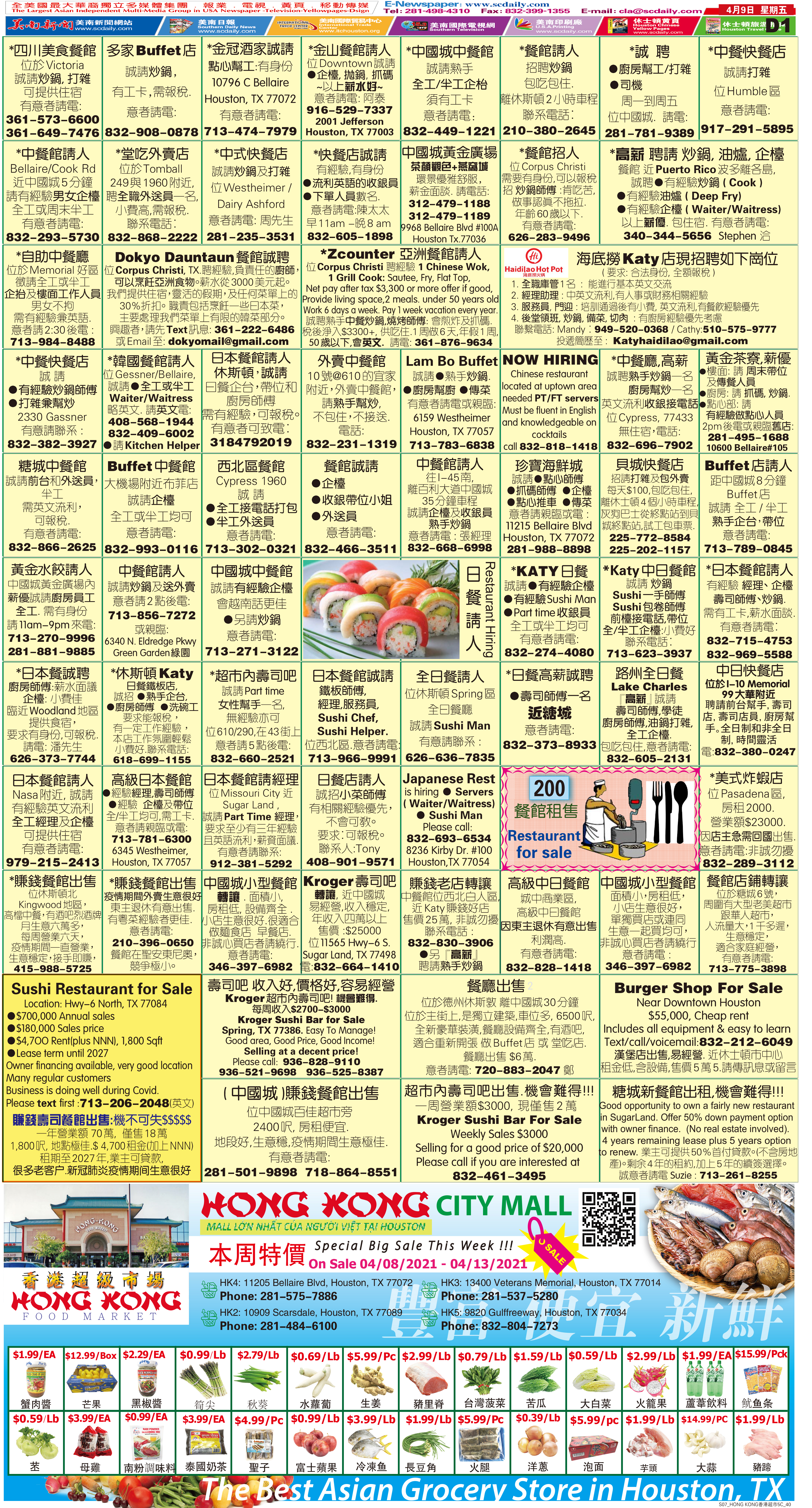210416 Epaper

 A Section B SectionC Section D Section210415 Epaper

 A Section B SectionC Section D Section210414 Epaper

 A Section B SectionC Section D Section210413 Epaper

 A Section B SectionC Section D Section210412 Epaper

 A Section B SectionC Section D Section210411 Epaper

 A Section B SectionC Section D Section210410 Epaper

 A Section B SectionC Section D Section210409 Epaper

 A Section B SectionC Section D Section210407 Epaper

 A Section B SectionC Section D Section210408 Epaper

 A Section B SectionC Section D Section210406 Epaper

 A Section B SectionC Section D Section